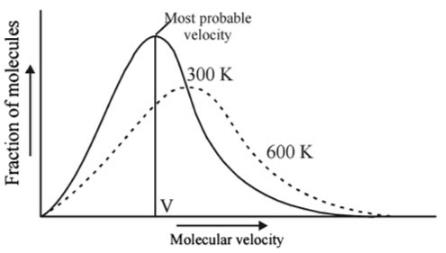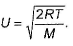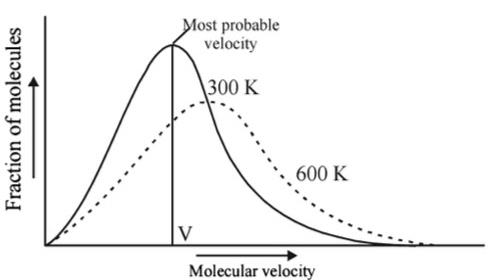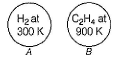Courses

# Test: Kinetic Molecular Theory

## 19 Questions MCQ Test Chemistry for JEE | Test: Kinetic Molecular Theory

Description
This mock test of Test: Kinetic Molecular Theory for JEE helps you for every JEE entrance exam. This contains 19 Multiple Choice Questions for JEE Test: Kinetic Molecular Theory (mcq) to study with solutions a complete question bank. The solved questions answers in this Test: Kinetic Molecular Theory quiz give you a good mix of easy questions and tough questions. JEE students definitely take this Test: Kinetic Molecular Theory exercise for a better result in the exam. You can find other Test: Kinetic Molecular Theory extra questions, long questions & short questions for JEE on EduRev as well by searching above.
QUESTION: 1

### Direction (Q. Nos. 1-11) This section contains 11 multiple choice questions. Each question has four choices (a), (b), (c) and (d), out of which ONLY ONE option is correct. Q. Helium atom is two times heavier than a hydrogen molecule (H2). At 298 K, the average kinetic energy of helium atom is

Solution:

Kinetic energy of gas molecule depends on temperature and does not depend upon the nature of gas.

K.E =(3/2)kT.

Thus hydrogen and helium have same K.E at same temperature.

QUESTION: 2

### Mass of gas is 300 gm and its specific heat at constant volume is 750J/kg K. if gas is heated through 75°C at constant pressure of 105 N/m2, it expands by volume 0.08 × 106 cm3. find CP/CV.

Solution:

Data given;
ΔT = 75 oC = 75 K
ΔV = 0.08 * 106 cm3 = 0.08 m3
Cv  = 750 J kg-1 K-1
m = 300 g = 0.300 kg
p  = 105 N m-2 = 100000 N m-2
The first law of thermodynamics;
ΔU = Q – W
Q = ΔU + W    ---- (1)
mCpΔT = mCvΔT + pΔV   ----- (2)
Dividing the above expression (2) with Cv , we get;
Cp / Cv = Cv / Cv + pΔV / mΔTCv
Cp / Cv = 1 + pΔV / mΔTCv
Cp / Cv = 1 + ([100000 N m-2 . 0.08 m3] /                 [0.300 kg . 75 K .750 J kg-1K-1])
Cp / Cv = 1 + 0.474
Cp / Cv = 1.474 ; After rounding it off we get,
= 1.5 (appx)

QUESTION: 3

### At what temperature will the collision frequency in N2 be equal to that in He at 25°C if both gases are at a pressure of 1.00 bar collision diameter = 0.373 nm for N2 = 0.218 nm for He (Assume equal number of molecules per unit volume.)

Solution:

Collision frequency=RMSvelocity/mean free path= √3RT/M/kT/ √2σ2p

R=gas constant=8.314J/K/mol

T=temperature

M=molecular mass

K=boltzmann constant

P=pressure, σ=collision constant

Collision frequency of nitrogen/ Collision frequency of nitrogen=[√3RT/M]/[kT/√2 σ2p] nitrogen/[√3RT/M]/[kT√2 σ2p]helium

As the collision frequency is same

=>[√3RT/M]/[kT/√2 σ2p] nitrogen=[√3RT/M]/[kT√2 σ2p]helium

=>[√T/M/T/ σ2p] nitrogen=[√T/M/T/ σ2p] helium

=> [σ2/M√T] nitrogen=[[σ2/M√T]helium

=0.373/18x√T=0.218/4x√298

=>T=17.80K

QUESTION: 4

According to the kinetic theory of gases, in an ideal gas, between two successive collisions a gas molecule travels

[IIT JEE 2003]

Solution:

According to kinetic theory of gases, a gas molecule travels in a straight line path.

QUESTION: 5

As the tem perature is raised from 20°C to 40°C. The average kinetic energy of the neon atoms changes by a factor of which of the following?

[IIT JEE 2004]

Solution:

Average kinetic energy ∝ Temperature in Kelvin

(KE40) / (KE20) = (3/2 nR * 313)/(3/2 nR*293)

KE40 / KE20 = T2/T1 = 40 + 273 / 20 + 273  = 313/293

QUESTION: 6

Which one of the following statements is not true about the effect of an increase in temperature on the distribution of molecular speeds in a gas?

[IIT JEE 2005]

Solution:

Distribution of molecular velocities at two different temperature is shown below:-At higher temperature, moree molecules have higher velocities and less molecules have lower velocities.
From the above figure, it is clear that with the increase in temperature, most probable velocity increases but the fraction of such molecules decreases,
So option c is correct.

QUESTION: 7

Bond energy of H2 gas is 104 kcal mol-1. The temperature at which average kinetic energy of gaseous H2 molecules is equal to energy required to dissociate the molecules into atoms, is

Solution:

Bond energy is the energy to break bonds and convert the sample into atoms.
We know that,
K.E of a mol of gas molecule = 3/2 RT
A/Q 3/2 RT = 104 kcal mol-1
3/2×8.314×T = 104×103 x 4.18 J
T = 34893 K

QUESTION: 8

Compressibility factor for CO2 at 400 K and 71.0 bar is 0.8697. Molar volume of CO2 under these conditions is

Solution:

We know that
Z = pVobserved /RT
0.8697   =   71×0.987×Vobserved / 0.0821×400
Vobserved = 0.8697×0.0821×400/71×0.987
= 0.406 L or 0.41 L

QUESTION: 9

At what temperature will the hydrogen molecules have the same kinetic energy per mole as nitrogen molecules at 280 K?

Solution:

KE = 3/2 nRT
for 1 mole KE=3/2 RT

It is independent of the molecular mass and constant for a particular temperature.

Therefore T will be same 280K

QUESTION: 10

Temperature at which average translation energy of a molecule is equal to the kinetic energy of the electron in first Bohr is orbit is

Solution:

Av. Translational Energy of a molecule= 3/2 KT -------(1)
Kinetic Energy of the electron in first Bohr's orbit= 13.6eV = 13.6×1.6×10-19J--------(2)
from eqn. 1&2
3/2KT=13.6×1.6×10-19
T = 1.05×105 K

QUESTION: 11

Pressure (p) of an ideal gas is related to kinetic energy (E) per unit volume by equation

Solution:

We know that
K.E = 3/2RT = 3/2pV
Dividing both sides by V
K.E/V = 3/2p
E = 3/2p     (here E is K.E per unit volume)
Or p = 2/3E

QUESTION: 12

Direction (Q. Nos. 12 and 13) This sectionis based on statement I and Statement II. Select the correct answer from the code given below.

Q.

Statement I : The pressure exerted by a gas is the result of collisions of the molecules with the walls of the container.

Statement II : Due to collision, momentum is changed and rate of change of momentum is equal to force.

Solution:

Both statements are correct and statement 2 is the correct explanation of statement 1. The collisions of the molecules among themselves and with the walls of the container are perfectly elastic. Therefore, momentum and kinetic energy of the molecules are conserved during collisions.

QUESTION: 13

Statement I : As velocity increases, distribution of molecules increases and is maximum at most probable velocity, after which distribution of molecules decreases.

Statement II : Most probable velocity is given bySolution:

Both statements are correct but statement 2 is not the correct explanation of 1.Velocity distribution follows this graph and not the most probable formula.

*Multiple options can be correct
QUESTION: 14

Direction (Q. Nos. 14-15) This section contains 4 multiple choice questions. Each question has four choices (a), (b), (c) and (d), out of which ONE or  MORE THANT ONE  is correct.

Q. If a gas is expanded at constant temperature

Solution:

At constant temperature, P is inversely proportional to V. So for expansion, V increases and so P decreases. KE is 3/2 kT (for one atoom) or 3/2RT(for 1 mole). And with constant temperature, KE also remains constant.

*Multiple options can be correct
QUESTION: 15

According to kinetic theory of gases

[ITT JEE 2011]

Solution:

According to me, all statements are correct.
As per the postulates of the kinetic theory of gases, the molecules of gas collide with each other and with the walls of the container. These collisions are perfectly elastic in nature.
The gas molecule with mass m and speed u collides with the walls of the container to transfer the momentum Δp=−2mu. Thus heavier molecules transfer more momentum to the walls of the container. But this is not the postulate of the kinetic theory of gases.
As per Maxwell -Boltzmann distribution of molecular speed, very few molecules move with very high or very low speed. Most of the molecules move with average speed. Hence, only a small number of molecules have very high
velocity.
According to the kinetic theory of gases, a gas molecule moves in straight line with constant velocity. When it collides with another molecule, it changes direction. Between collisions, the molecules move in straight lines with constant velocities.

QUESTION: 16

Direction (Q. Nos. 16-19) This section contains a paragraph, wach describing theory, experiments, data etc. three Questions related to paragraph have been given.Each question have only one correct answer among the four given options (a),(b),(c),(d).

Passage I

Volume occupied by molecules is negligible compared to total volume of molecules. We have N2 gas molecules assuming them spherical of radius 200 pm.

Q.
Total volume of molecules contained in one mole of the gas is

Solution:

Volume of single molecule = 4/3π(r)3
= 4/3×π×(200×10-12)3 m3
V = 3.349×10-29 m3
Volume of 1 mole (NA molecules) =  3.349×10-29 m3×6.022×1023
= 2.016×10-5 m3
= 2.016×10-5×106 cm3 or 20.16 cm3

QUESTION: 17

Passage I

Volume occupied by molecules is negligible compared to total volume of molecules. We have N2 gas molecules assuming them spherical of radius 200 pm.

Q. Percentage of empty space available at STP is

Solution:

volume of one molecule of Nitrogen = 4/3πr3
= 4/3 x 3.14 x ( 2 x 10-10)3
= 3.351 x 10-29 m3
volume of one mole of N2 molecules
= 3.351 x 10-29 x 6.023 x 1023 m3
= 2.018 x 10-5 m3
volume of one mole of N2 gas = 22.4 Litre at NTP = 22.4 x 10-3 m3
space occupied by the molecules = 2.018x10-5/22.4x103x100
= 0.09 %
Empty space = 100 - 0.09 = 99.91 %

QUESTION: 18

Passage lI

Two flasks A and B have equal volumes as shown Both gases occupy 22.40 dm3 at STP. Also collision diameter of C2H4 molecule is four times than that of H2 molecule.Q. In which flask and how many times are molecules moving faster?

Solution:

(VAvg)a = √(8RT/πM)
On potting the value of condition of A and B, we get
VH2 = k(12.24)
VC2H4 = k(5.65)
Thus, molecules in H2 are moving 2.16 times faster.

QUESTION: 19

Passage lI

Two flasks A and B have equal volumes as shown Both gases occupy 22.40 dm3 at STP. Also collision diameter of C2H4 molecule is four times than that of H2 molecule.Q. In which flask and how many times is the mean free path of the molecules greater?

Solution: